# Recognizing Handwritten Digits with scikit-learn

Data analysis is not limited to numbers and strings, because images and sounds can also be analyzed and classified. Recognizing handwritten text is a problem that can be traced back to the first automatic machines that needed to recognize individual characters in handwritten documents.

To address this issue in Python, the scikit-learn library provides a good example to better understand this technique, the issues involved, and the possibility of making predictions. scikit-learn python library is commonly used in solving machine learning and data science problem from the begging to the end. scikit-learn built on the machine learning libraries.

.Numpy

.Scipy

.Matplotlib

scikit-learn offers an extensive is range of built in algorithms that make the most of data science projects: here are the main ways the scikit-learn library is used.

Let’s start,

## Today we going to implement a digit recognizer. The Digits data set of the scikit-learn library provides numerous data-sets that are useful for testing many problems of data analysis and prediction of the results. Some Scientist claims that it predicts the digit accurately 95% of the times. Perform data Analysis to accept or reject this Hypothesis.

#importing the dataset

# Print to show there are 1797 images (8 by 8 images for a dimensionality of 64)
print(“Image Data Shape” , digits.data.shape)

import numpy as np
import matplotlib.pyplot as plt
import warnings
warnings.filterwarnings(“ignore”)

plt.figure(figsize=(20,4))
for index, (image, label) in enumerate(zip(digits.data[0:5], digits.target[0:5])):
plt.subplot(1, 5, index + 1)
plt.imshow(np.reshape(image, (8,8)), cmap=plt.cm.gray)
plt.title(‘Training: %i\n’ % label, fontsize = 20)

# Generating Model

## As we can clearly see for well above 95% of our models the achieved accuracy is 100% . Hence we can easily conclude that our model works for more than 95% of the time.

import matplotlib.pyplot as plt
import seaborn as sns
from sklearn import metrics
cm = metrics.confusion_matrix(y_test, predictions)
plt.figure(figsize=(9,9))
sns.heatmap(cm, annot=True, fmt=”.3f”, linewidths=.5, square = True, cmap = ‘Pastel1’);
plt.ylabel(‘Actual label’);
plt.xlabel(‘Predicted label’);
all_sample_title = ‘Accuracy Score: {0}’.format(score)
plt.title(all_sample_title, size = 15);

# Conclusion:

Here we learn ,how to import dataset from sklearn,how to build model and make prediction using estimator function fit() and predict() . we also calculate accuracy ,in short we introduced with Skleran library.

--

--

--

## More from Amit Jaiswal

Data Science Learner

Love podcasts or audiobooks? Learn on the go with our new app.

## The ML Surprise## I made a model! How do I use it? — Beginning Model Building, Part 1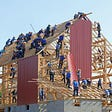## Tile-Coding: An Efficient Sparse-Coding Method for Real-Valued Data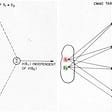## Model Building in B2B SaaS## Tax Payers Fake ID Card Detection | A Machine Learning Project Part 1## ElegantRL: Much Much More Stable than Stable-Baseline3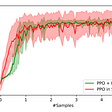## No-Frills Guide to Passing the AWS Certified Machine Learning Specialty Exam## Amit Jaiswal

Data Science Learner

## How to Make Systematic Choices of Machine Learning Models## Different Normalization Techniques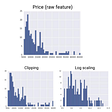## Supervised learning Vs unsupervised learning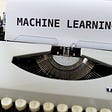## Fake Job prediction with Machine Learning